# How to convert time to decimal number, hours, minutes or seconds in Excel

The tutorial demonstrates different ways to convert time to decimal in Excel. You will find a variety of formulas to change time to hours, minutes or seconds as well as convert text to time and vice versa.

Because Microsoft Excel uses a numeric system to store times, you can easily turn hours, minutes and seconds into numbers that you can use in other calculations.

In general, there are two ways to convert time to decimal in Excel - by changing the cell format and by using arithmetic calculations or Excel time functions, such as HOUR, MINUTE and SECOND. Further on in this tutorial, you will find the detailed explanation of the first way and formula examples demonstrating the other technique.

## How to convert time to decimal number in Excel

Overall, there are three ways to change a time value to a decimal number: arithmetic operation, CONVERT function or a combination of three different Time functions.

The easiest way to convert time to decimal in Excel is to multiply the original time value by the number of hours, seconds or minutes in a day:

• To convert time to a number of hours, multiply the time by 24, which is the number of hours in a day.
• To convert time to minutes, multiply the time by 1440, which is the number of minutes in a day (24*60).
• To convert time to seconds, multiply the time time by 86400, which is the number of seconds in a day (24*60*60 ).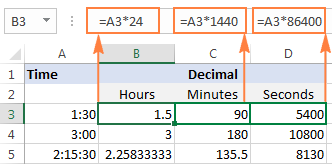In the following sections, you will learn the other methods of converting times to a decimal number in Excel.

### How to convert time to hours in Excel

This section demonstrates 3 different formulas to convert hours from the standard time format (hh:mm:ss) to a decimal number.

#### Formula 1: Arithmetic calculation

You already know the fastest way to convert a time value to a number of hours in Excel - multiplying by 24, i.e. by the number of hours in one day:

`=A2*24`

Where A2 is the time value.

To get the number of compete hours, embed the above formula in the INT function that will get rid of the fractional part:

`=INT(A2*24)`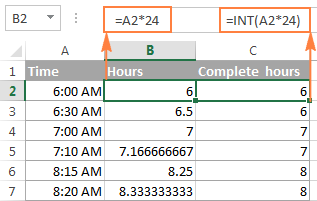#### Formula 2: CONVERT function

Another way to perform the "time > hours" conversion is to use the following Convert formula:

`=CONVERT(A2, "day", "hr")`

#### Formula 3: HOUR, MINUTE and SECOND functions

Finally, you can use a bit more complex formula, whose logic, however, is quite obvious. Extract the individual time units by using the HOUR, MINUTE and SECOND functions, then divide minutes by 60 (the number of minutes in an hour) and seconds by 3600 (the number of seconds in an hour), and add up the results:

`=HOUR(A2) + MINUTE(A2)/60 + SECOND(A2)/3600`

### How to convert time to minutes in Excel

The same three methods can be used to convert minutes from the standard time format to a decimal number.

#### Formula 1: Arithmetic calculation

To convert time to total minutes, you multiply time by 1440, which is the number of minutes in one day (24 hours * 60 minutes = 1440):

`=A2*1440`

If you want to return the number of compete minutes, utilize the INT function like in the previous example:

`=INT(A2*1440)`

You can view the results in the screenshot below: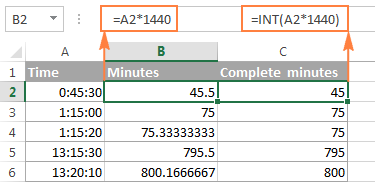#### Formula 2: CONVERT function

To do the "time > minutes" conversion with the CONVERT(number, from_unit, to_unit) function, supply "day" as the unit to convert from and "mn" as the unit to convert to:

`=CONVERT(A2, "day", "mn")`

#### Formula 3: HOUR, MINUTE and SECOND functions

One more way to get the number of minutes is to multiply hours by 60 and divide seconds by the same number:

`=HOUR(A2)*60 + MINUTE(A2) + SECOND(A2)/60`

### How to convert time to seconds in Excel

Converting time to total seconds in Excel can be done in a similar fashion.

#### Formula 1: Arithmetic calculation

Multiply the time value by 86400, which is the number of seconds in a day (24 hours * 60 minutes * 60 seconds = 86400):

`=A2*86400`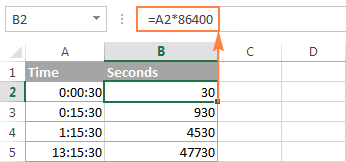#### Formula 2: CONVERT function

The formula is basically the same as in the above examples with the only difference that you convert the "day" unit to "sec":

`=CONVERT(A2, "day", "sec")`

#### Formula 3: HOUR, MINUTE and SECOND functions

Use the HOUR, MINUTE and SECOND functions like in the two previous examples (I believe at this point you don't need any further explanation of the formula's logic :)

`=HOUR(A2)*3600 + MINUTE(A2)*60 + SECOND(A2)`

Tips:

1. If any of the above formulas returns a value formatted as time, simply change the cell's format to Generalto display it as a number.
2. To convert time to a decimal number that represents the time in the internal Excel system, apply the General format to the cell. With this approach, 23:59:59 will be converted to 0.99999, 06:00 AM to 0.25, and 12:00 PM to 0.5. If an integer part of the converted number is greater than zero, it means that your cell contains both date and time values.

## How to split date and time in Excel

As is often the case, your Excel worksheet may contain dates and times in one cell, while you want to split them into two separate cells.

Remembering that in the internal Excel system the date value is stored as a whole part and the time value as a fractional part of a decimal number, you can extract the date using the INT function, which rounds the cell value down to the nearest integer.

Supposing your original dates and times are in column A, the following formula will accomplish the separation:

`=INT(A2)`To extract the time portion, subtract the date returned by the above formula from the original date and time value:

`=A2-B2`

Where column A contains the original date & time values and column B contains the dates returned by the INT function.

If you'd rather not have time values linked to the separated dates (for example, you may want to remove the date column in the future), you can use the following MOD formula that refers to the original data only:

`=MOD(A2,1)`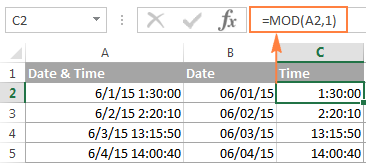Tip. If the separated date and time values are not displayed properly, change the format of the new columns to Date and Time, respectively.

This is how you split date and time in Excel. If you want to further separate hours, minutes and seconds into individual columns, then use the HOUR, MINUTE and SECOND functions, as demonstrated in How to get hours, minutes and seconds from a timestamp.

## How to spell time in Excel

Sometimes, you may need to convert time into the format that reads something like "# days, # hours, # minutes and # seconds". A good thing is that you already know all the ingredients of the formula:

• Extract days using the INT function;
• Extract time units with HOUR, MINUTE and SECOND functions, and
• Concatenate all parts in a single formula.

Having difficulties with figuring out a proper formula for your worksheet? The following example will make things easy!

Supposing you have the dates of upcoming events in column B beginning in cell B4, and the current date and time returned by the NOW() function in cell B1.

The formula to calculate the time difference is as simple as `=B4-\$B\$1`. Of course, nothing prevents you from subtracting the current date and time directly with `=B4-NOW()`.

And now, let's make a countdown timer that would show how many days, hours, minutes and seconds are left until each event.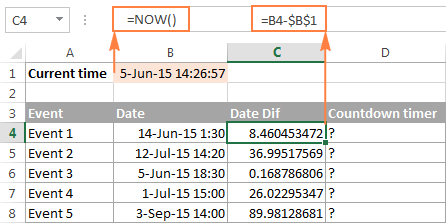The formula to enter in cell D4 is as follows:

`=INT(C4) & " days, " & HOUR(C4) & " hours, " & MINUTE(C4) & " minutes and " & SECOND(C4) & " seconds"`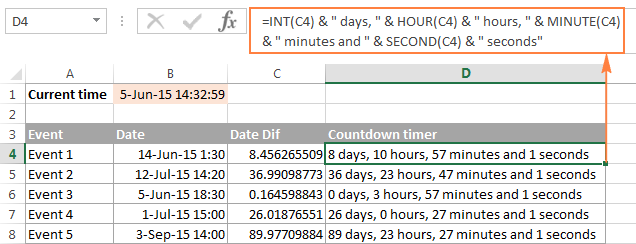If you wish to get rid of 0 values, like in cells D6 and D7 in the screenshot above, then include the following IF statements:

`=IF(INT(C4)>0, INT(C4)&" days, ","") & IF(HOUR(C4)>0, HOUR(C4) & " hours, ","") & IF(MINUTE(C4)>0, MINUTE(C4) & " minutes and ","") & IF(SECOND(C4)>0, SECOND(C4) & " seconds","")`

All zeros are gone!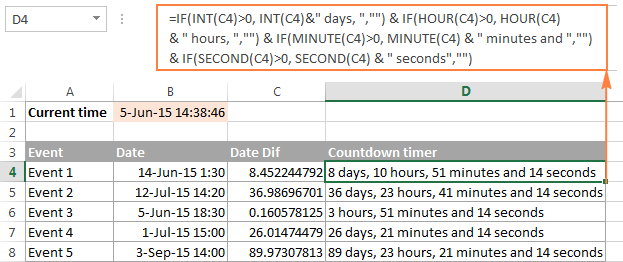Note. When either of the above formulas refers to a negative number, the #NUM! error will appear. This may happen when you subtract a bigger time from a smaller one.

An alternative way to write time in words in Excel is to apply the following custom time format to the cell: d "day," h "hours," m "minutes and" s "seconds". No formulas and no calculations are required! For more information, please see Creating a custom time format in Excel.

## Convert text to time in Excel

If your time formulas and calculations do not work right, time values formatted as text is often the cause. The fastest way to convert text to time in Excel is using the TIMEVALUE function.

The Excel TIMEVALUE function has just one argument:

TIMEVALUE(time_text)

Time_text is a text string in any of the time formats that Excel can recognize. For example:

`=TIMEVALUE("6:20 PM")`

`=TIMEVALUE("6-Jan-2015 6:20 PM")`

`=TIMEVALUE(A2)` where A2 contains a text string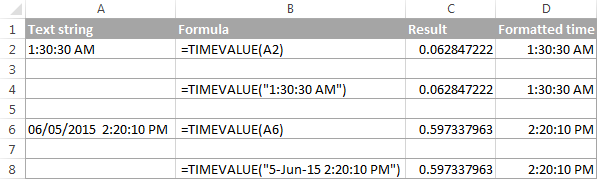As you see, the formulas with cell references and corresponding text strings deliver identical results. Also, please notice the left alignment of time strings (text values) in cells A2 and A6 and right-aligned converted time values in column D.

## Convert time to text in Excel

Supposing you have an Excel file full of times formatted to look like "8:30:00 AM" and you want to convert them to the text format. Simply changing the cell's format to TEXT won't work because this would change your time values to underlying numeric representation of the time. For example, 8:30:00 AM will be converted to decimal 0.354166666666667.

So, how do you convert cells to the text format so that your cells still have the time in them? The answer is to use the TEXT function that converts a numeric value to text with the display formatting that you specify, for example:

`=TEXT(\$A2,"h:mm:ss")`

The screenshot below demonstrates other possible formats: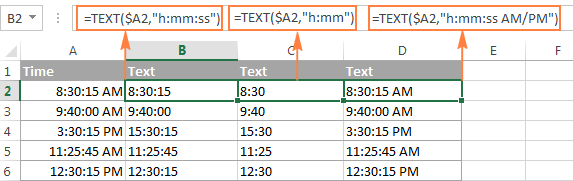## How to convert numbers to time format in Excel

If you have a list of numbers such as 1, 2, 3.5 and you want to convert them to a time format, for example 1:00:00, 2:00:00 or 3:30 AM, perform the following steps.

1. Divide the numbers by 24 (there are 24 hours in a day). The formula can be as simple as =A2/24.
2. Select the cell(s) with the formula result, right-click and select Format Cells from the context menu or press Ctrl+1. Either way, the Format Cells dialog will appear, you select Time on the left pane under Category, and choose the format you want on the right pane under Type. Please see How to format time in Excel for more details.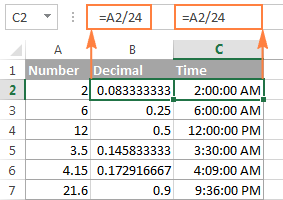If your time exceeds 24 hours, 60 minutes, 60 seconds, use these guidelines to correctly show it.

That's all for today. If someone wants to get the first-hand experience with the formulas discussed in this article, you are most welcome to download the sample workbook below.

If you want to learn a few more useful formulas to calculate times in Excel, please check out the related tutorials at the end of this page. I thank you for reading and hope to see you again next week!

Converting time in Excel - examples (.xlsx file)

## Excel Times tutorials

1. Alex,

I have over 2500 records. I used the formula above to calculate the difference in dates, converted to days (=F3-\$C\$3).

Converted time (Sep 27, 2023 07:04 PM) - Created time (Sep 25, 2023 08:32 AM) and have the answer of 2.44 which is great.

However, when I try to click and drag, the first part of the formula changes, but not the second. Dragging from (=F3-\$C\$3), looking for (=F4-\$C\$4) but getting (=F4-\$C\$3).

I have tried to do multiple cells (up to 10) but get the same type of result (=F14-\$C\$4), (=F15-\$C\$5). where the second part of the formula just repeats the previously highlighted fields, instead of updating similar to the first part of the formula.

If you could help, it would be greatly appreciated.

2. Hi Alex, - i have used the formula =MOD(G192-F192,1)*24 to make the total working hours - his total working time came as 12.32, but actual calculation as per the time is he worked for 12 hrs and 19 min, pls suggest the formula what i can use the round down below 25 minutes and round up 25 minutes as an .30 minutes and above 45 minutes 1 hour in single formula.

• 12.32 is the time 12 hrs and 19 min, written as a decimal number. You can read about this in the article above. To get the number of minutes, use the MINUTE function. Use the rounding functions to round the time up or down. For example,

=IF(MINUTE(A1)<25, FLOOR(A1,"1:00"), IF(MINUTE(A1)<45, FLOOR(A1,"0:30"), CEILING(A1,"1:00")))

3. 02:59:18
03:59:18
04:11:00
04:33:13
05:01:56
05:01:58

i can pivot table. But i didn't proper result in pivot table. I have drag time column to column in pivot table. it's show time only. but i need Hour,Minutes .

Pivot table properly working: i have select time column any one cell i have click f2 + Enter key for all time column cell it's will be working...

• Sorry, it's not quite clear what you are trying to achieve.

4. Source I want
7:09:30 7.9
7:02:50 7.2

• See the answer in the first paragraph of the article above

5. Hi,

I want this 176 Hours 06 Mins

In Numbers like - 176.06

• Hi! To extract numbers from text and insert a dot between them, try this formula:

=SUBSTITUTE(TRIM(CONCAT(IF(ISNUMBER(--MID(A1,ROW(\$1:\$95),1)),MID(A1,ROW(\$1:\$95),1)," ")))," ",".")

6. Hi, would everyone know how to conver this time stamp from SAP business objects report?

"Updated
Time"
102752
103343
114240
120446
125726
143743
144708
145859
150353
155545
160009
160009

• I can't guess what result you want to get. I’ll try to offer you the following formula:

=TIME(LEFT(A1,2),MID(A1,3,2),RIGHT(A1,2))

7. Hello
Am trying to convert hours in this format 150:00:00 to format 150 ,is it possible or it can only be done manually?

• Hi! Above in the article it is written that to convert hours into numbers, you must multiply by 24. Read it carefully.

8. Hello, thank you so much for this, below formula is what i need but in case i want to deduct the number of weekends in it, is it possible?

=INT(C4) & " days, " & HOUR(C4) & " hours, " & MINUTE(C4) & " minutes and " & SECOND(C4) & " seconds"

im thinking if incorporating networkdays formula but i was not able to get the result i want

• Hi! You cannot use the NETWORKDAYS function to find the difference in hours and minutes because it only works with days. If I have misunderstood, please clarify your question.

9. May 1 2023 1:03AM
May 1 2023 7:30AM
Convert to M/DD/YYYY HH:MM:SS AM/PM format

• Hi! If your date is written as text, use the formula below to convert it to date and time. Then apply the date and time custom format you want.

=DATE(MID(A1,FIND("~",SUBSTITUTE(A1," ","~",2),1)+1,4), VLOOKUP(LEFT(A1,3), {"JAN",1;"FEB",2;"MAR",3;"APR",4;"May",5;"JUN",6;"JUL",7;"AUG",8;"Sep",9;"OCT",10;"NOV",11;"DEC",12},2,0), MID(A1,FIND(" ",A1,1)+1,2)) + TIMEVALUE(MID(A1,LEN(A1)-6,5)&" "&RIGHT(A1,2))

10. I have a cell with numbers in the format of YYYYMMDDHHMMSS (20230501080229), need to convert to YYYY MM DD HH MM SS (2023 May 01 08:02:29) to easily read on the spreadsheet. Thank you.

• Hi! Use the MID function to extract the required digits and create the date and time using the DATE and TIME functions.

=DATE(MID(A1,1,4),MID(A1,5,2),MID(A1,7,2))+TIME(MID(A1,9,2),MID(A1,11,2),MID(A1,13,2))

Set the date and time format you want in the cell, for example "yyyy mmmm dd hh:mm:ss"

11. 2023-03-01T00:16:36+00:00
2023-03-01T00:35:39+00:00
2023-03-01T00:39:00+00:00
2023-03-01T01:01:44+00:00
2023-03-01T01:05:57+00:00
2023-03-01T01:25:51+00:00
2023-03-01T01:40:14+00:00
2023-03-01T01:55:27+00:00
2023-03-01T02:13:56+00:00
2023-03-01T02:27:51+00:00
2023-03-01T02:31:06+00:00
2023-03-01T02:33:34+00:00
2023-03-01T02:43:46+00:00
2023-03-01T02:52:38+00:00
2023-03-01T02:56:37+00:00
2023-03-01T02:57:09+00:00
2023-03-01T03:05:35+00:00
2023-03-01T03:08:44+00:00
2023-03-01T03:14:07+00:00
2023-03-01T03:20:39+00:00
2023-03-01T03:33:29+00:00
2023-03-01T03:43:00+00:00
2023-03-01T03:54:52+00:00
2023-03-01T04:01:29+00:00
2023-03-01T04:12:33+00:00
2023-03-01T04:16:18+00:00
2023-03-01T04:21:23+00:00
2023-03-01T04:27:26+00:00
2023-03-01T04:34:44+00:00

I want to convert these timestamps into hourly basis

• Hi! Your question is not very clear. Give an example of what result you want to get.

12. Got time in decimal form and want 15,3722222222222 and want to form it into minutes and hours, tried =TEXT(A1/24;"h:mm:ss") with result: h:22:20 what am I doing wrong?

• Hi! I got the right result. Check your regional settings. Maybe your hours are not marked with "hh" but with something else.

13. Hello,

I feel like I've tried everything. I have a report that needs to be converted to total minutes. See data example below.

1d 05h 44m
6h 16m
3h 00m
0m
1h 02m
1d 04h 36m

I need to convert it to show the following in total minutes. Most of the formulas I've tried error when there is a cell that has only "m" or "h" and "m' data.

1784
376
180
0
62
1716

Is that possible?

Thanks!

14. I have a column of Time say as below and rows with name. The format says General. I now wanted to show the same in a pivot but in Pivot its taking as 0:00:00 and not showing as follows. I have 3 ppl working on different days and some days its zero so wanted to know which day they had come and which day they didnt. Basically pivot is not capturing the time.

03:59:13
09:14:04
08:36:58
08:01:34

15. I'm trying to convert 2 minutes & 30 seconds in excel from this format 2:30 into a decimal which should show as 2.5 minutes.
Another example is 15 seconds currently shows as 0:15 in excel and I need it to show it as 0.25 minutes.
How do I do that?

• Hi!
If these values are written as time and a custom time format (e.g. mm:ss) is used, use the instructions in the first paragraph of this article: How to convert time to decimal number in Excel.

16. i have wind data each 12 second and i wanted to change it to each 1 second with interpolation
TIME WIND SPEED
12:33:05 2
12:33:10 2
12:33:15 1
12:33:21 1
12:33:26 1
12:33:31 2
12:33:36 2
12:33:41 2
12:33:46 1
12:33:51 1
i need to change it to second time appreciate your help

17. Hello, I am setting up a payroll calculator on excel and wanted to see if there is an if function or any function that I can use to calculate payroll correctly. I current input hours formatted as text and then multiply by 24 but It gets thrown off if there is a whole number.

• i have data sheet for wind turbine in every 10 second i get wind speed, and i need to resample this data in every second (The model is
based on a 1 s sample time, in order to resample the wind speeds the 10 s data was interpolated linearly between points)
T W
12:33:05 2
12:33:10 2
12:33:15 1
12:33:21 1
12:33:26 1
12:33:31 2
12:33:36 2
12:33:41 2
12:33:46 1
12:33:51 1
12:33:56 1
12:34:01 1
12:34:06 1
12:34:11 1
12:34:16 1
12:34:21 1
12:34:26 1
12:34:31 1
12:34:36 1
12:34:41 1
12:34:46 2
12:34:52 2
EXPECTED LIKE THIS
12:33:05 2
12:33:06 2.1
12:33:07 2.15
12:33:08 1.09
12:33:09 1.51
12:33:10 2
12:33:11 2
12:33:12 2
12:33:13 1
12:33:14 1
12:33:15 1
12:34:16 1
12:34:17 1
12:34:18 1
12:34:19 1
12:34:20 1
12:34:21 1
12:34:22 1
12:34:23 1
12:34:24 1
12:34:25 2
12:34:26 2

18. I need to convert numbers to hours and mins
2.03 is what I have in excel I need 2:03 is there away to format the data

• Hi!
Replace the separator "." with ":" in the number using the SUBSTITUTE function. Use the TIMEVALUE function to convert text to time, as described above.

19. thank you! i was struggling for an hour to Sum a lot of work hours on a spreadsheet, converting the AM/PM hours with INT() then doing SUM() worked great!

20. Hi!

I would like to get your best guess on how to have a cycle time of a task calculated in the right way: data in one (!) cell is like "2 Days 5.49 Hours" as one example.

I now would like to have following information: How many business hours does it take for a colleague to finish this job ?

21. I would like to change the time from e.g. 1h 32m 4s to 01:32:04 without having to do it individually. How would I do this?

• Hello!
Extract the numbers from the text and use the TIMEVALUE function to convert the text to time. Try the formula:

=TIMEVALUE(SUBSTITUTE(TRIM(CONCAT(IF(ISNUMBER(--MID(H1,ROW(\$1:\$30),1)),MID(H1,ROW(\$1:\$30),1)," ")))," ",":"))

• hello dear,

its work but once i have a time as a "01h 31sec " its dropped with wrong time
how can be flexed becouse the time fromat that im tried to fixed it like this
15h 30m 20s
01h 30s

• Hi!
I was unable to reproduce your problem. For these values, the formula works for me.

• Its really work, bu unfortunately once i have the value as ( 01h 22sc ) its dropped

• Hi!
The formula doesn’t work since it has been created based on the details you provided in your first query. I see from your subsequent comment that your task differs from the one you originally described.
If the time is not written down completely, the formula will not help.

22. 1 days 2 hours 20 minutes How to convert it into 26 hours 20 minutes
I tried but result is not yet got

• Set up the number format to custom like that: [h]:mm

23. I am trying to show total hours as days and hours with this formula as it shows Days hours and minutes which is what I need.
=INT(C4) & " days, " & HOUR(C4) & " hours, " & MINUTE(C4) & " minutes and " & SECOND(C4) & " seconds"
In C6 I have 40.00 hours carried over in column F6 is 4.0 hours extra holiday
I add the hours together in H6 to show a total of 44 hours
In column I have H6/8 = 5.5 days (working an 8-hour day).
The formula shows this as 5 days 12 hours 0 min 0 sec.
I understand that it sees the .5 as half a day - 12 hours.
Is there a way to convert this so it shows as 5 days 4 hours.
I have tried changing H6 to divide by 24 and have tried H6 to divide by 3.
Is what I am asking possible.

Regards
Peter

24. Hi all,

could I possibly get some pointers on the below:

i usually download a report with a time in and time out for our engineers on site.
is there an easy way to calculate if they have been on site from 7:30 am to 5:30 pm to get a full shift.
the shifts are based on a 10 hour work day.
for example if they work 9h45m - they would get 0.97 of a shift.

this would save me days of work! i'd appreciate any help!

Many thanks!!

25. Hello

I would like to calculate the lateness

Time in should be 830 = 8:30am ( I use =TIME(IF(LEN(A1)=2,0,LEFT(A1,LEN(A1)-2)),RIGHT(A1,2),0) , this formula provided by you )
Actual in 903 = 9:03am
How to prepare formula for lateness in minutes ? and show in minutes ?

• Hi!
Calculate the time using this formula in two cells and subtract time. Or subtract in one formula

=TIME(IF(LEN(A2)=2,0,LEFT(A2,LEN(A2)-2)),RIGHT(A2,2),0) - TIME(IF(LEN(A1)=2,0,LEFT(A1,LEN(A1)-2)),RIGHT(A1,2),0)

26. I have a spreadsheet that we use and the "time" is listed as a custom "time" using the format 00":"00, which would show 1:30 Pm as 13:30

I need to convert that "time" to an actual time string for 24 hour clock using an excel formula but I cannot figure it out. Can you assist??

• Hi!
Unfortunately, I don't quite understand your problem. Try using a custom time format. If this does not help, explain the problem in detail.

27. hi,

i tried using 'To convert the time to decimal, multiply it by 24' but it didn't work, is there another method ?

thanks

• Hi!
The information you provided is not enough to understand your case and give you any advice, sorry.

28. Hi,

Is there any formula in Excel which it could directly convert day/hour/minute display into total minutes?

Example: 1d 20h = 2640 mins

Thanks!

29. Good evening,
Maybe you can help me out, it would be so great. And I'm sorry if you've already directly answered this question. I couldn't find it. I work on a 24 hour schedule and need to figure out to track my hours. For example, I come in at 1305 and leave at 1535. I am looking for a formula that will enter the time I worked in decimal hours so equaling 2.50 hours, not in total time (2:30) . I can compute it by hand of course, but who wants to do that? Haha thank you very much for your help!

• Hi!
To convert the time to decimal, multiply it by 24. See the first paragraph of the article above.

30. Hello Sir,

I would like you to help me to sort out this small issue which I’m unable to do here is my problem

Example
4534HRS - 2449SEC (I need total hours here)
It do repeats daily like initial and final

4530HRS - 2343SEC 4533HRS - 975SEC
4533HRS - 975SEC 4534HRS - 2449SEC

Kindly help me to fix this issue.Thanks in advance

31. I am trying to convert time on duty as follows in one cell to a number of hours in another
8:00-16:00 - is that possible?
Thank you
Grainne

• Hello!
Extract the text before and after "-" delimiter using these guidelines: Split string by delimiter or pattern, separate text and numbers.
Convert text to time using the TIMEVALUE function. Calculates the difference between the specified times, as described in this article.

=(TIMEVALUE(MID(A1,SEARCH("-",A1)+1,50)) - TIMEVALUE(LEFT(A1,SEARCH("-",A1)-1)))*24

If you need to convert the time to a number, multiply by 24.

32. Hi,

I am trying to convert 3.50 to show 3hours and 50 minutes in time format.
Working out new hours for a store allocated for a service, the current hours are formatted to a decimal place for 3hrs 50 minutes. Whereas the way I have calculated it was 4hr 15 minutes in the H:MM format. How can I get this to translate the same for the 3.50, as I need to find the variation in time format.

For example: old hours 3hr 50 mins, new hours 4hr 15mins = an uplift of 25minutes. I have tried to convert the 3.50 to the H:MM format is just gives me 12pm or 84:00. Which is wrong.

New Hours Current hours
4:15 3.50

• Hello!
Replace "," with ":" and use the TIMEVALUE function to get the time.

=TIMEVALUE(SUBSTITUTE(A1,",",":"))

Don't forget to set the time format for the cell with the formula.

• I just get the value error.

• Hi!
Use the SUBSTITUTE function to replace the decimal separator in the number with ":". If it is a dot, use "." in the formula. instead of ","

33. We bill by hours and tenths so I need a formula to convert the result to hours and tenths by set minute increments as follows:
• 1-6 = 0.1
• 7-12 = 0.2
• 13-18 = 0.3
• 19-24 = 0.4
• 25-30 = 0.5
• 31-36 = 0.6
• 37-42 = 0.7
• 43-48 = 0.8
• 49-54 = 0.9
• 55-60 = 1.0
2:34 should output 2.6; 1Hr 5 minutes = 1.1; 2 Hrs 15 minutes = 2.3; 3 hrs 45 minutes = 3.8
Is there a formula that will auto calculate the end result to hours and tenths? Up until now I have done that field manually...

• Hello!
The answer to your question can be found in the article above. Read it carefully. Use the rounding functions if necessary.

=ROUND(A1*24,1)

34. Hi i want to calculate time worked on excel to be converted into hours worked. but time is filled in one cell only eg 12:45-13:00 and the next cell answers .25 hours worked ?

Can you assist?

• Hi!
Extract the time from the text using the LEFT and RIGHT functions and convert it to time using TIMEVALUE function, as described in the article above. Read carefully.

=TIMEVALUE(LEFT(A1,5))

=TIMEVALUE(RIGHT(A1,5))

35. How do I convert Actual elapsed time "5311" to 1 Hour 28 Minutes using a formula

• Hi!
Sorry, it's not quite clear what you are trying to achieve.
Why is “5311” equal to 1 Hour 28 Minutes?

36. I have the following in a spread sheet and am wondering how I go about setting up a formula to learn the difference between the cells. It is a HUGE data sheet with almost 20k entries. The day as just a number and not a full date is throwing me off.

Day Hour:Min:Sec

29 18:25:26 29 18:39:12

• The data points should have looked like this
29 18:25:26
29 18:39:12

37. How do I go from 0800-1200 to 8:00am-12:00pm In One cell

38. I always search here when I stuck in any excel issue and I have never been disappointed. Keep up the good work.

39. Hi, I have a dataset with time being in the General number format. I know I can manually select the cell to be formatted in time, but don't want to do it manually for all the rows. Can someone tell me how the conversion works? Thanks

For example:
44653.8395833333 is equivalent to 4/2/2022 8:09:00 PM

40. Hello, I have an excel sheet with the time in format "11 Days 2 Hours 58 Min 0 Sec" I want to convert this into either a numeric format or just into minutes. What formula could I use to accomplish this?

41. Good morning. I am trying to count the presence of specific digits *within* a column of time values. For example, how many minutes in a column of times end in the character 0? I can't find that anywhere online, and experimenting with COUNT, COUNTIF and COUNTIFS gives me obviously bad answers. Please help?

• Hello!
Convert a time value to text with the TEXT function. Then extract the last digit using the RIGHT function and count matches with "0".
The formula below will do the trick for you:

=SUM(--(RIGHT(TEXT(A1:A10,"hh:mm"),1)="0"))

• Thank you. I think we're a lot closer to success, but I'm getting a #VALUE! error with that.

I used TEXT function to convert the time column to a column of pure text that looks like it should, basically 1746 rows of values like this...

18:01
18:48
20:15
20:45
21:35
21:59
22:10
22:30
22:59
23:32
05:30

That worked. Then I pasted your formula, modified for all the row numbers in column E containing the data...

=SUM(--(RIGHT(TEXT(e3:e1748,"hh:mm"),1)="0"))

...and got the #VALUE! error. If you can help, I'd hugely appreciate!

• Hi!
Unfortunately, I was unable to reproduce your issue. The formula is designed to work with time values, but it can also work with times written as text. With the data you provided, it also works correctly.

42. Dear Sir,
I have to compare two time sets and check which of them appeared earlier.
so using the delta between TIME A and TIME B:
DELTA = TIME A - TIME B

if Delta is >0, than no problem, i will get the time difference and can be transformed to Text, etc. in order draw an average.
BUt, if Delta is <0, i have a problem because (TIME*24) is not working if the Time is a negative number.

I hope my explanation is clear.
regards,

43. If I need to have the clock in times and clock out times to be converted to decimal values that consist of 0-4 minutes = 0 , 4-9 =.1, minutes, can the formula be set up to do the conversions for me?

• Hi!
Have you tried the ways described in this blog post? If they don’t work for you, then please describe your task in detail, I’ll try to suggest a solution.

44. Hello,

I was wondering how to translate a time written as a whole number to a time value. For example, the values I have on my spreadsheet are values listed as "543" for 5:43 AM and "1350" for 1:50 PM. I also have values that are "50" for 12:50 AM. Can you please help me translate these values into a time value?

Thank you

• Hello!
To create a time value, use the TIME function.

=TIME(IF(LEN(A1)=2,0,LEFT(A1,LEN(A1)-2)),RIGHT(A1,2),0)

45. Hello,

I am struggling with something that might be simple so I apologize in advance if it is.

I'll give an example:
it takes 00h 00min 03sec to bake a cookie
I want to know how much time it will take to bake 500k cookies.
so I want excel to multiply 00h 00min 03sec with 500k
The result should be same format 00h 00min 03sec. I also need to be able to convert the hours in days if needed.

Jamie

• Hi!
If I understand your task correctly, try the following custom time format:

"0h 0min" [ss]"sec"

Hope this is what you need.

46. Hello, I am working as a logistics coordinator and i have 2 questions:

1) If i have a driver who finishes driving at 18:00(F7) and starts next day at 06:00(E8) it means he has 12hrs of rest time. I used formula =24-F7+E8. But if on sunday he doesnt drive at all. For example finishes saturday at 14:00 but starts only monday at 06:00. What formula could i use to automatically add 24 if there is a blank cell?

2) Is it possible to use format not ''12:45'' but ''12h 45min'' when it counts the rest time?

Thank you

47. 10 Day(s) 22 Hr(s) 34 Min(s)

How to convert this into days.

• Hi!
To convert hours to days, divide by 24. To convert minutes to days, divide by 1440.

• Hi Sir , Thanks for the response.

i have tried that the same but not getting the exact days.

10 Day(s) 22 Hr(s) 34 Min(s) - I want to convert this in days only , like 10days 22 hrs should come as 11 days. seeking for your help on this.
Thank You